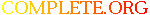Complete.Org: Mailing Lists: Archives: freeciv-dev: November 2001: [Freeciv-Dev] Re: topology RFC (again)# [Freeciv-Dev] Re: topology RFC (again)

[Top] [All Lists]

 To: jdorje@xxxxxxxxxxxx Cc: freeciv-dev Subject: [Freeciv-Dev] Re: topology RFC (again) From: "Ross W. Wetmore" Date: Wed, 07 Nov 2001 00:30:54 -0500

```Some pseudo code examples to help explain key points once again.
i.e. the arithmetic to do isometric (un)normalizations :-?

Cheers,
RossW
=====

At 04:45 PM 01/11/02 -0500, Jason Dorje Short wrote:
>"Ross W. Wetmore" wrote:
[...]
>> You may have to be clever to figure out just how you need to represent
>> this wrapping or unwrapping algorithm. It is actually the same thing.
>> If you feed in (0,0) for (x0,y0) for the rectangular case this should
>> be clear, as it reduces cleanly to the simple normalize we have become
>> accustomed to. When you work out the (un)normalize_iso_pos() they
>> should have similar relationships that you can use to check.
>
>No.  unnormalize_map_pos(&x, &y, 0, 0) is not the same thing as
>normalize_map_pos(0, 0).  Normalize map_pos wraps (x, y) into the normal
>set, which need not correspond to any particular representative set that
>unnormalize_map_pos wraps into.

Jason, THE normal set N(0,0) is something one designates in the manner
needed. Once one has defined it, say to reference real memory in some
appropriate manner, this is what normalize_map_pos() is written to
return. unnormalize_map_pos() given the appropriate offset values will
clearly return the offsets in this set if one chooses.

This is not a technical issue. Just some stupid interpretation you can't
seem to let go of. Unfortunately for you, the above definitions provide
quite a useful way to understand and deal with these issues, whereas
your interpretation doesn't help things much.

>Take a look at the normalize_map_pos_[iso|rect] from my "general
>topology" patch.  (Aside from the width/height parameters, these are the
>equivalent of your unnormalize_map_pos function.)  Although we're just
>doing simple linear-algebra operations, the arithmetic is very, very
>ugly.  And the implementation isn't even correct (it doesn't fit the
>"wrap-to-a-square" criteria specified above).

Actually, it isn't. For one thing, you have replaced all the while's with
if's, so your version won't work with anything not a wrap distance from
your N(0,0) set. We'll leave the iso problems till a later analysis. You
can judge the degree of ugliness below for yourself.

>So, you're right.  To implement unnormalize_map_pos you will have to be
>clever and very good at arithmetic.

Ok, lets fix up the unnormalize function for iso keeping things in
rectangular map coordinates. There is probably a condition on the
wrapping vectors that they be orthogonal to use this algorithm.

THE normalized set or N(0,0) is defined by the rectangle formed by
the wrapping vectors (i.e. vector dimensions of the rectangle) at
some offset of your choice. (map.ysize,0) conveniently keeps all
positions of this normalized set at small positive position. Note,
just because we used (0,0) for the simple rectangular N(0,0) doesn't
restrict us to use some other origin when working with iso.

Note as well, this still defines a very practical unnormalization that
is useful for accessing memory or describing the full iso GUI in its
standard form or any subset of this (after appropriate transformations).
This is what the current rectangular GUI gives, so it is perfectly
adequate for isometric play. It also guarantees tiles are only shown
once. We'll deal with your odd GUI tastes in a later discussion.

#define DOT(u,v)        (u*v + u*v)
#define ADD(t,u,v)      (t= u + v, t= u + v)
#define SUB(t,u,v)      (t= u - v, t= u - v)

struct topo {
int  origin;   /* map offset of THE normalized set reference point */
int  xwrap;    /* vector for xwrapping operation */
int  ywrap;    /* vector for ywrapping operation */
int  area;        /* total tile count of a normalized set if needed */
} mapiso = {
{map.ysize, 0},             /* 0, 0          for rectangular */
{map.xsize, map.xsize},     /* map.xsize, 0                  */
{-map.ysize, map.ysize},    /* 0, map.yzize                  */
map.xsize*map.ysize*2       /* map.xsize*map.ysize           */
};

/* iso-rectangular (un)normalize (in map coordinates)
*
* This finds the point in the iso-rectangular normalized set at
* offset {x0,y0}.
*
* Note: normalize_mapiso_pos() is just this function called with
*       {x0,y0} = mapiso.origin.
*/
unnormalize_mapiso_pos(&un_x, &un_y, x0, y0) {
int nn, tt, vv, v0;

/* unnormalize is only valid for real coordinates */
if( !is_real_tile(*un_x, *un_y) )
return FALSE;

/* unnormalize vv to an iso-rectangle with origin at v0 */
vv= *un_x, vv= *un_y;
v0= x0, v0= y0;

if( IS_WRAP_ISO_X ) {
/* Ignoring denominator in unit vectors mapiso.xwrap/|mapiso.xwrap| */
nn = DOT(v0, mapiso.xwrap);
while( DOT(vv, mapiso.xwrap) < nn )

nn = DOT(t, mapiso.xwrap);
while( DOT(vv, mapiso.xwrap) >= nn )
SUB(vv, vv, mapiso.xwrap);
}

if( IS_WRAP_ISO_Y ) {
/* Ignoring denominator in unit vectors mapiso.ywrap/|mapiso.ywrap| */
nn = DOT(v0, mapiso.ywrap);
while( DOT(vv, mapiso.ywrap) < nn )

nn = DOT(t, mapiso.ywrap);
while( DOT(vv, mapiso.ywrap) >= nn )
SUB(vv, vv, mapiso.ywrap);
}

/* vv currently holds the offset into the iso-rectangle     */
/* compute map coordinates for this iso set, i.e. origin v0 */
/* Note: if v0 == mapiso.origin, this is THE normalized set */
*un_x= vv, *un_y= vv;

return TRUE;
}
====

/* Simple rectangular (un)normalize
*
* This finds the point in the map.xsize x map.ysize normalized set
* at offset x0, y0. It is just an optimized version of the above.
*
* Note: normalize_map_pos() is just this function called with (0,0)
*/
unnormalize_map_pos(&un_x, &un_y, x0, y0) {

/* unnormalize is only valid for real coordinates */
if( !is_real_tile(*un_x, *un_y) )
return FALSE;

if( IS_WRAP_X ) {
while( *un_x < x0 )
*un_x += map.xsize;
while( *un_x >= x0 + map.xsize )
*un_x -= map.xsize;
}

if( IS_WRAP_Y ) {
while( *un_y < y0 )
*un_y += map.ysize;
while( *un_y >= y0 + map.ysize )
*un_y -= map.ysize;
}

*un_x = *un_x + x0, *un_y = *un_y + y0;

return TRUE;
}
====

```